• plt.show() 坐标轴右边和上边的边框去掉 #gca代表获取当前坐标轴 ax = plt.gca() ax.spines['right'].set_color('none') ax.spines['top'].set_color('none') #右边和上边的边框去掉 设置x轴，y轴位置，以及它们的...
import numpy as np

设置x,y范围
x = np.linspace(-3,3,100)
y1 = 2*x + 1
y2 = x**2

#xy范围
plt.xlim((-1,2))
plt.ylim((-2,3))

plt.plot(x,y1,color='red',linewidth=1.0,linestyle='--')
plt.plot(x,y2,color='blue',linewidth=5.0,linestyle='-')
#color指的是线的颜色，linewidth指的是线的粗细程度
#linestyle指的是线的类型
#第一个两个-指的就是虚线，第一个一个-指的就是实线
plt.show()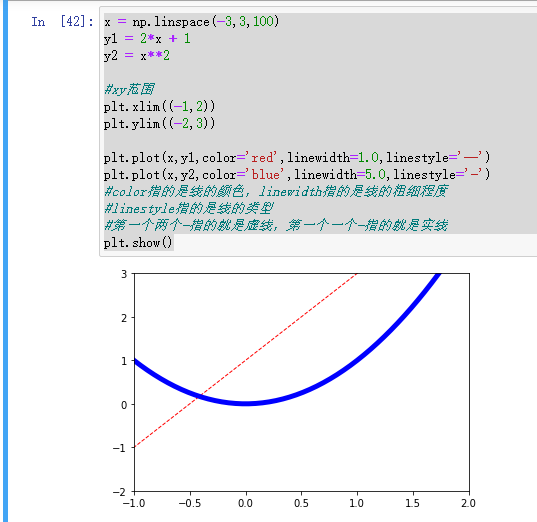对x，y轴进行描述
x = np.linspace(-3,3,100)
y1 = 2*x + 1
y2 = x**2

#xy范围
plt.xlim((-1,2))
plt.ylim((-2,3))

#xy描述
plt.xlabel('xxxx')
plt.ylabel('yyyy')

plt.plot(x,y1,color='red',linewidth=1.0,linestyle='--')
plt.plot(x,y2,color='blue',linewidth=5.0,linestyle='-')
#color指的是线的颜色，linewidth指的是线的粗细程度
#linestyle指的是线的类型
#第一个两个-指的就是虚线，第一个一个-指的就是实线
plt.show()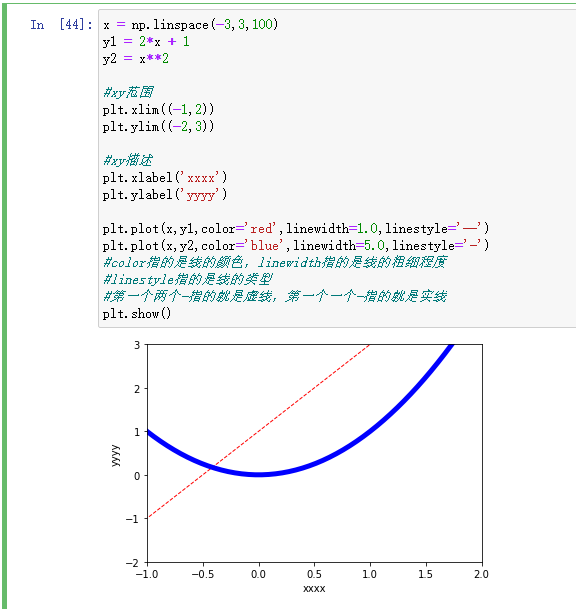将x轴坐标自定义一组数据，y轴改为文字的
new_ticks = np.linspace(-2,2,11)
print(new_ticks)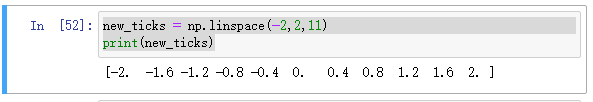x = np.linspace(-3,3,100)
y1 = 2*x + 1
y2 = x**2

#xy范围
plt.xlim((-1,2))
plt.ylim((-2,3))

#xy描述
plt.xlabel('xxxx')
plt.ylabel('yyyy')

plt.plot(x,y1,color='red',linewidth=1.0,linestyle='--')
plt.plot(x,y2,color='blue',linewidth=5.0,linestyle='-')
#color指的是线的颜色，linewidth指的是线的粗细程度
#linestyle指的是线的类型
#第一个两个-指的就是虚线，第一个一个-指的就是实线

plt.xticks(new_ticks)
plt.yticks([-1,0,1,2,3],
['level1','level2','level3','level4','level5'])
plt.show()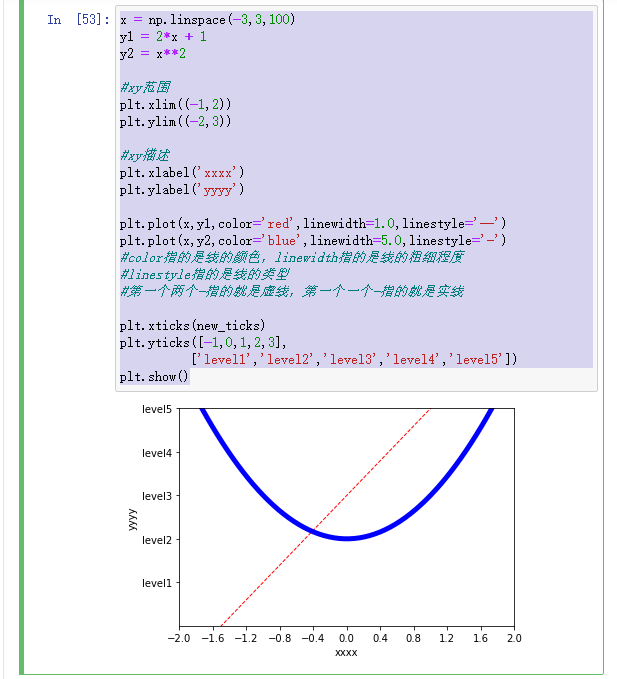设置坐标轴颜色
new_ticks = np.linspace(-2,2,11)
print(new_ticks)

x = np.linspace(-3,3,100)
y1 = 2*x + 1
y2 = x**2

#xy范围
plt.xlim((-1,2))
plt.ylim((-2,3))

#xy描述
plt.xlabel('xxxx')
plt.ylabel('yyyy')

plt.plot(x,y1,color='red',linewidth=1.0,linestyle='--')
plt.plot(x,y2,color='blue',linewidth=5.0,linestyle='-')
#color指的是线的颜色，linewidth指的是线的粗细程度
#linestyle指的是线的类型
#第一个两个-指的就是虚线，第一个一个-指的就是实线

plt.xticks(new_ticks)
plt.yticks([-1,0,1,2,3],
['level1','level2','level3','level4','level5'])

#gca代表获取当前坐标轴
ax = plt.gca()
ax.spines['right'].set_color('red')
#右边的边框设置为红色
ax.spines['top'].set_color('red')
#上面的边框也设置为红色

plt.show()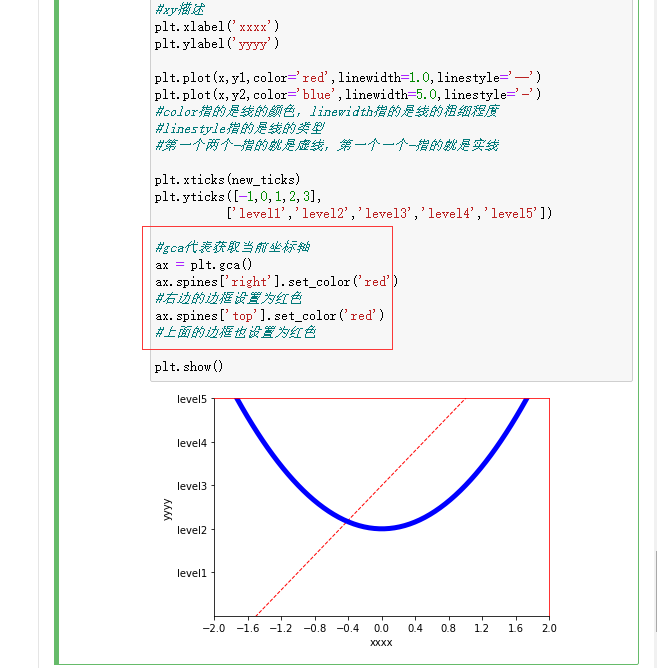坐标轴右边和上边的边框去掉

#gca代表获取当前坐标轴
ax = plt.gca()
ax.spines['right'].set_color('none')
ax.spines['top'].set_color('none')
#右边和上边的边框去掉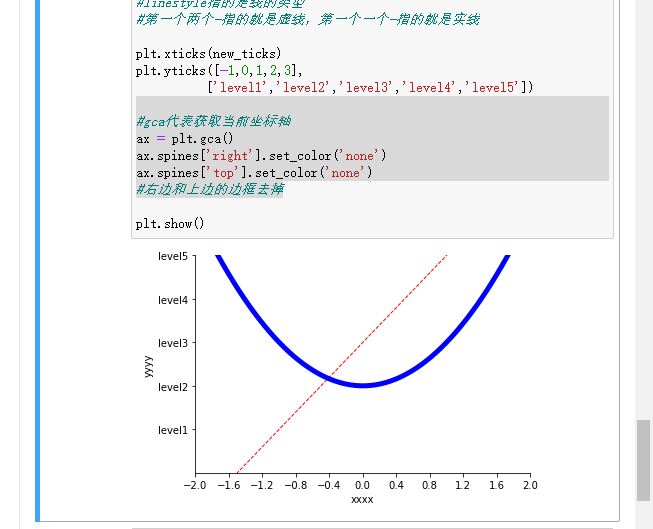设置x轴，y轴位置，以及它们的刻度位置
import matplotlib.pyplot as plt
import numpy as np

new_ticks = np.linspace(-2,2,11)
print(new_ticks)

x = np.linspace(-3,3,100)
y1 = 2*x + 1
y2 = x**2

#xy范围
plt.xlim((-1,2))
plt.ylim((-2,3))

#xy描述
plt.xlabel('xxxx')
plt.ylabel('yyyy')

plt.plot(x,y1,color='red',linewidth=1.0,linestyle='--')
plt.plot(x,y2,color='blue',linewidth=5.0,linestyle='-')
#color指的是线的颜色，linewidth指的是线的粗细程度
#linestyle指的是线的类型
#第一个两个-指的就是虚线，第一个一个-指的就是实线

plt.xticks(new_ticks)
plt.yticks([-1,0,1,2,3],
['level1','level2','level3','level4','level5'])

#gca代表获取当前坐标轴
ax = plt.gca()
ax.spines['right'].set_color('none')
ax.spines['top'].set_color('none')
#右边和上边的边框去掉

#把x轴的刻度设置为'bottom'，x轴刻度上的字都在x轴下部
#把y轴的刻度设置成'left'，y轴刻度上的字都在y轴左部
ax.xaxis.set_ticks_position('bottom')
ax.yaxis.set_ticks_position('left')

#设置x,y轴位置，让x轴y轴位于0的位置
ax.spines['bottom'].set_position(('data',0))
ax.spines['left'].set_position(('data',0))
plt.show()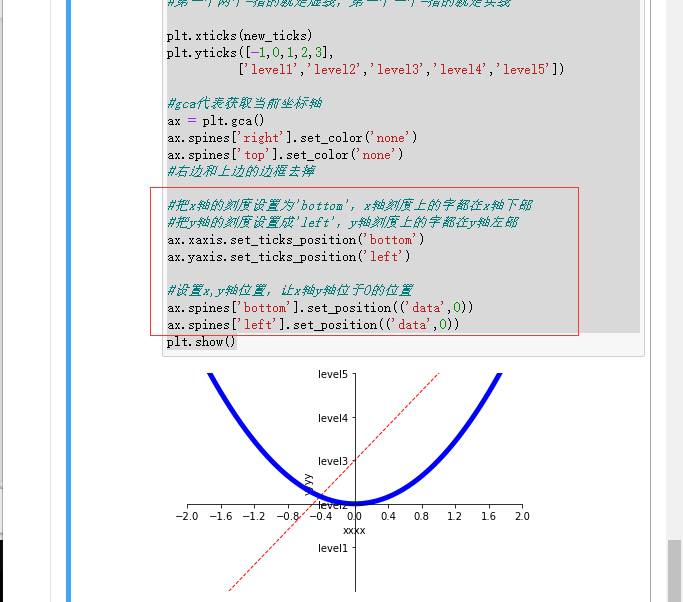展开全文python
• 使用import导入模块matplotlib.pyplot，并简写成plt 使用import导入模块numpy，并简写成np import matplotlib.pyplot as plt import numpy as np 使用np.linspace定义x：范围是(-3,3);个数是50. 仿真一维数据组(x ,...
调整名字和间隔
使用import导入模块matplotlib.pyplot，并简写成plt 使用import导入模块numpy，并简写成np
import matplotlib.pyplot as plt
import numpy as np

使用np.linspace定义x：范围是(-3,3);个数是50. 仿真一维数据组(x ,y1)表示曲线1. 仿真一维数据组(x ,y2)表示曲线2.
x = np.linspace(-3, 3, 50)
y1 = 2*x + 1
y2 = x**2

使用plt.figure定义一个图像窗口. 使用plt.plot画(x ,y2)曲线. 使用plt.plot画(x ,y1)曲线，曲线的颜色属性(color)为红色;曲线的宽度(linewidth)为1.0；曲线的类型(linestyle)为虚线.
plt.figure()
plt.plot(x, y2)
plt.plot(x, y1, color='red', linewidth=1.0, linestyle='--')

使用plt.xlim设置x坐标轴范围：(-1, 2)； 使用plt.ylim设置y坐标轴范围：(-2, 3)； 使用plt.xlabel设置x坐标轴名称：’I am x’； 使用plt.ylabel设置y坐标轴名称：’I am y’；
plt.xlim((-1, 2))
plt.ylim((-2, 3))
plt.xlabel('I am x')
plt.ylabel('I am y')
plt.show()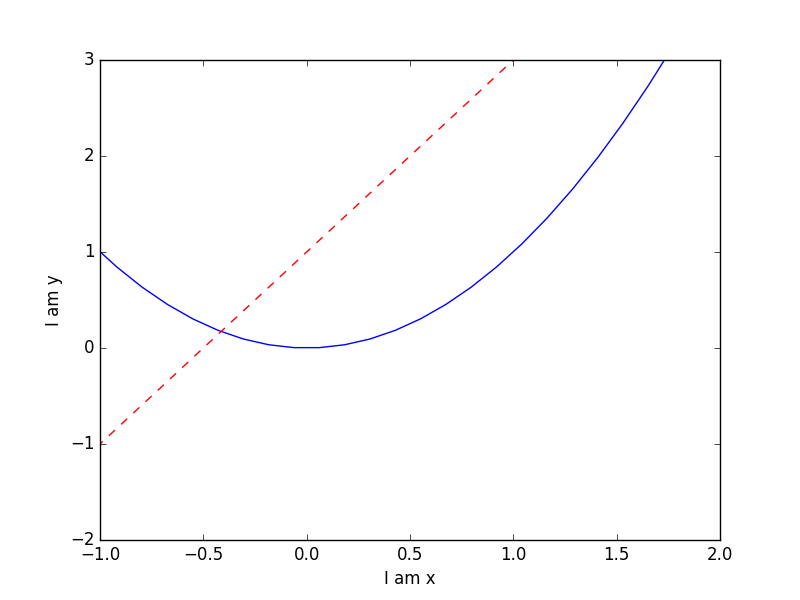使用np.linspace定义范围以及个数：范围是(-1,2);个数是5. 使用print打印出新定义的范围. 使用plt.xticks设置x轴刻度：范围是(-1,2);个数是5.
new_ticks = np.linspace(-1, 2, 5)
print(new_ticks)
plt.xticks(new_ticks)

plt.yticks([-2, -1.8, -1, 1.22, 3],[r'$really\ bad$', r'$bad$', r'$normal$', r'$good$', r'$really\ good$'])
plt.show()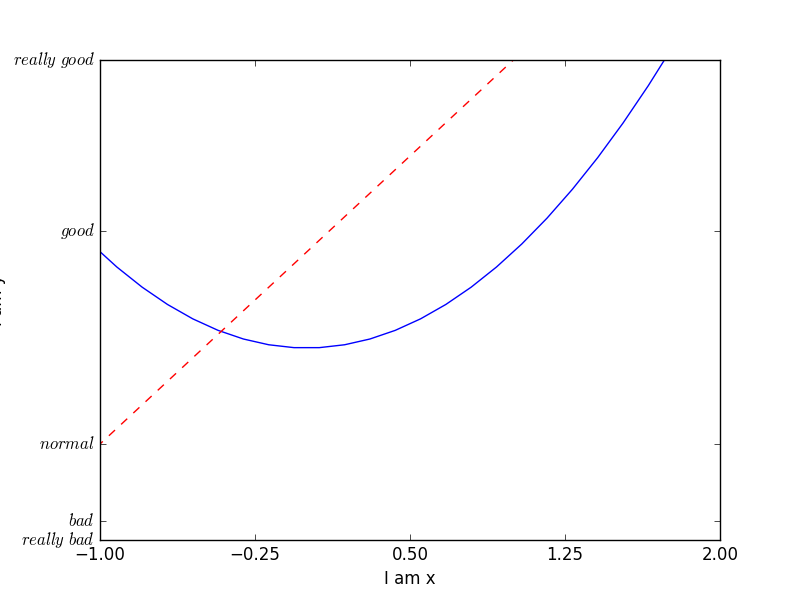设置不同名字和位置
使用import导入模块matplotlib.pyplot，并简写成plt 使用import导入模块numpy，并简写成np
import matplotlib.pyplot as plt
import numpy as np

使用np.linspace定义x：范围是(-3,3);个数是50. 仿真一维数据组(x ,y1)表示曲线1. 仿真一维数据组(x ,y2)表示曲线2.
x = np.linspace(-3, 3, 50)
y1 = 2*x + 1
y2 = x**2

使用plt.figure定义一个图像窗口. 使用plt.plot画(x ,y2)曲线. 使用plt.plot画(x ,y1)曲线，曲线的颜色属性(color)为红色;曲线的宽度(linewidth)为1.0；曲线的类型(linestyle)为虚线. 使用plt.xlim设置x坐标轴范围：(-1, 2)； 使用plt.ylim设置y坐标轴范围：(-2, 3)；
plt.figure()
plt.plot(x, y2)
plt.plot(x, y1, color='red', linewidth=1.0, linestyle='--')
plt.xlim((-1, 2))
plt.ylim((-2, 3))

new_ticks = np.linspace(-1, 2, 5)
plt.xticks(new_ticks)
plt.yticks([-2, -1.8, -1, 1.22, 3],['$really\ bad$', '$bad$', '$normal$', '$good$', '$really\ good$'])

使用plt.gca获取当前坐标轴信息. 使用.spines设置边框：右侧边框；使用.set_color设置边框颜色：默认白色； 使用.spines设置边框：上边框；使用.set_color设置边框颜色：默认白色；
ax = plt.gca()
ax.spines['right'].set_color('none')
ax.spines['top'].set_color('none')
plt.show()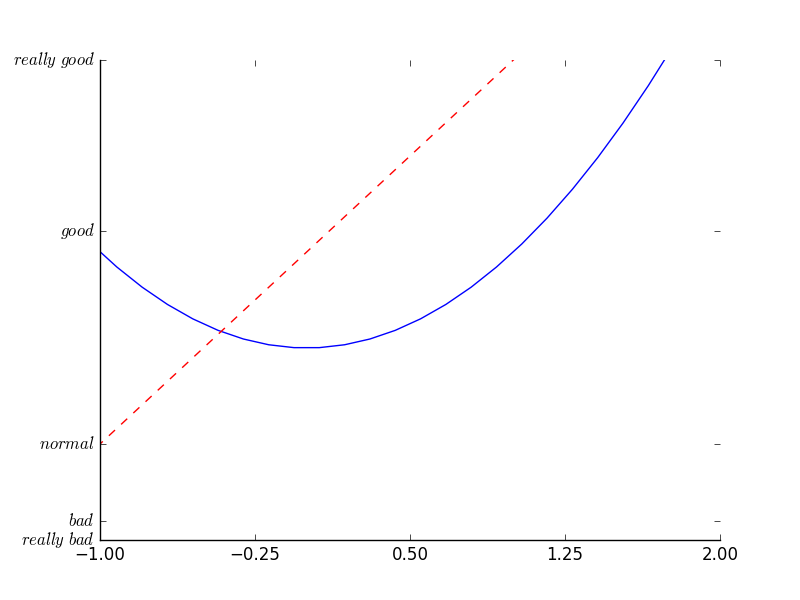调整坐标轴
使用.xaxis.set_ticks_position设置x坐标刻度数字或名称的位置：bottom.（所有位置：top，bottom，both，default，none）
ax.xaxis.set_ticks_position('bottom')

使用.spines设置边框：x轴；使用.set_position设置边框位置：y=0的位置；（位置所有属性：outward，axes，data）
ax.spines['bottom'].set_position(('data', 0))
plt.show()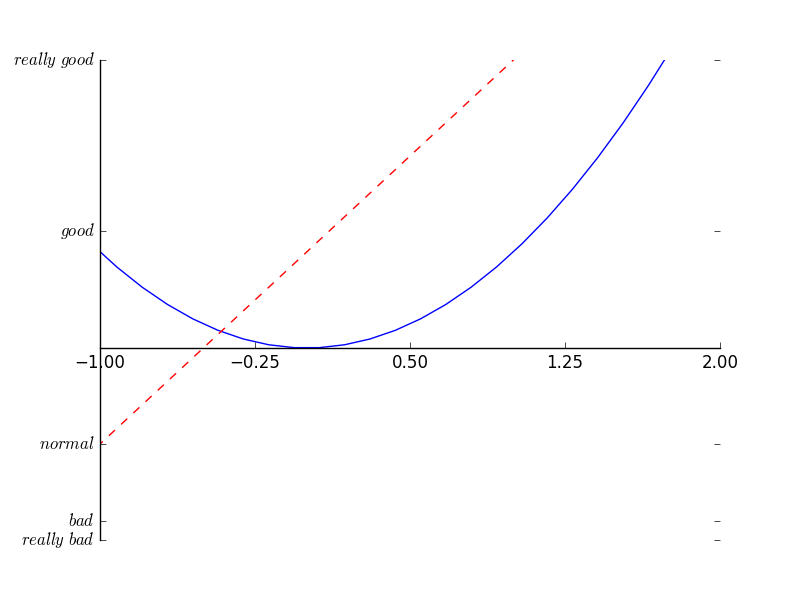使用.yaxis.set_ticks_position设置y坐标刻度数字或名称的位置：left.（所有位置：left，right，both，default，none）
ax.yaxis.set_ticks_position('left')

使用.spines设置边框：y轴；使用.set_position设置边框位置：x=0的位置；（位置所有属性：outward，axes，data） 使用plt.show显示图像.
ax.spines['left'].set_position(('data',0))
plt.show()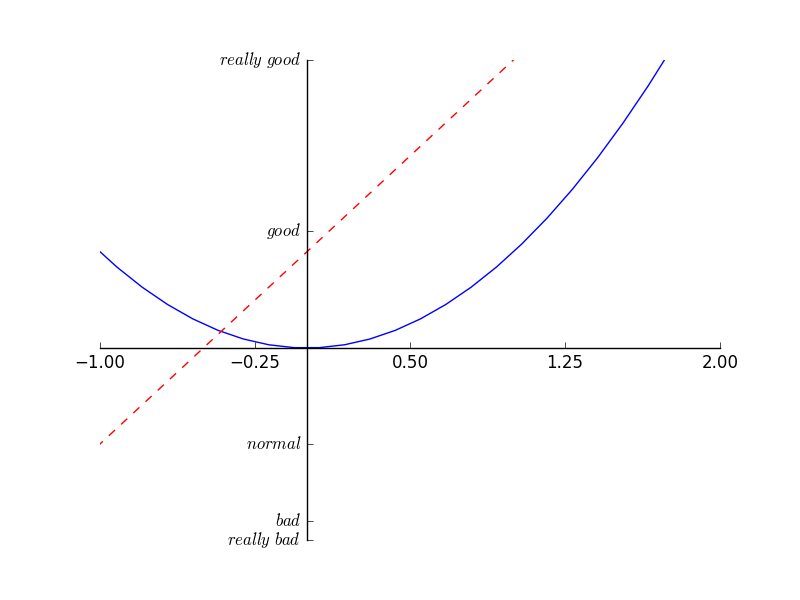展开全文• python如何获取matplotlib图中的坐标轴标签get_yticklabels from matplotlib import pyplot as plt fig = plt.figure(figsize=(16,12), dpi=(300)) ax1 = fig.add_subplot(111) p = ax1.bar(range(5), range(5)) ...


python如何获取matplotlib图中的坐标轴标签get_yticklabels

from matplotlib import pyplot as plt

fig = plt.figure(figsize=(16,12), dpi=(300))
p = ax1.bar(range(5), range(5))
plt.draw()

>>> [item.get_text() for item in ax1.get_yticklabels(which='both')]
['0.0', '0.5', '1.0', '1.5', '2.0', '2.5', '3.0', '3.5', '4.0']

展开全文python 机器学习
• matplotlib，利用pyplot与面向对象的api两种方式设置坐标轴刻度的个数以及标签
这里介绍两种设置坐标轴刻度的方法，一种是利用pyplot提交的api去进行设置，另一种是通过调用面向对象的api， 即通过matplotlib.axes.Axes去设置。
第一种方式： 通过pyplot进行设置，通过pyplot下的xticks进行设置，这个api的原型如下：
matplotlib.pyplot.xticks(ticks=None, labels=None, **kwargs)

ticks：array-like, optional 表示xtick 位置列表，传递一个空列表会删除所有 xticks。
labels：array-like, optional 要放置在给定刻度ticks位置的标签。仅当也传递刻度ticks时才能传递此参数。
其他常用的参数： rotation：设置坐标轴标签的倾斜角度 fontsize: 设置坐标轴标签的字体的大小
例如：
import matplotlib.pyplot as plt

fig = plt.figure()
ticks = [1, 2, 3]  # 指定坐标轴上进行显示的刻度（坐标轴默认的刻度为[0, 0.2, 0.4, 0.6, 0.8, 1.0]）
labels = [2012, 2013, 2014]  # 准备与上面指定的坐标轴的刻度对应替换的标签列表
plt.xticks(ticks, labels, rotation=30, fontsize=15)  # 调用xticks进行设置
plt.show()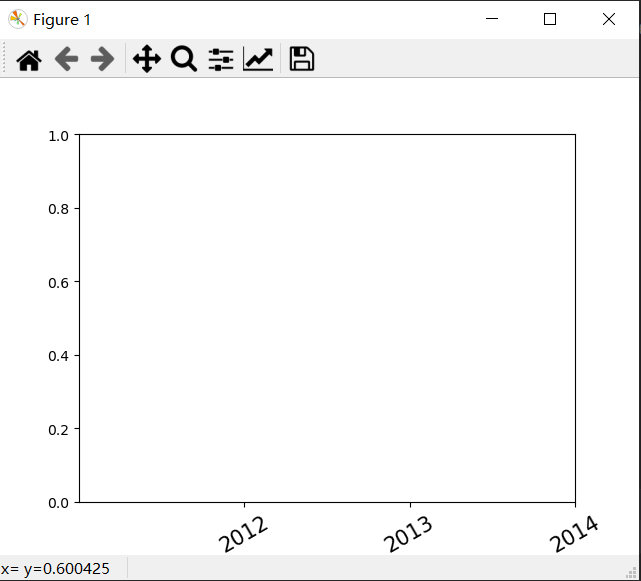第二种方式： 利用面向对象的api进行设置，首先用matplotlib.axes.Axes.set_xticks设置坐标轴的刻度，然后利用matplotlib.axes.Axes.set_xticklabels替换上面的刻度。
Axes.set_xticks(self, ticks, *, minor=False)

ticks: list of floats 这个参数表示要显示的坐标轴的刻度，需要传入list列表
Axes.set_xticklabels(self, labels, *, fontdict=None, minor=False, **kwargs)

labels: list of str 与上面set_xticks指定的坐标轴的刻度对应替换的标签列表
例子：
import matplotlib.pyplot as plt

fig, ax = plt.subplots()

ticks = [1, 2, 3]  # 指定坐标轴上显示的刻度（坐标轴默认的刻度为[0, 0.2, 0.4, 0.6, 0.8, 1.0]）
labels = [2012, 2013, 2014]  # 准备上面指定的坐标轴的刻度对应替换的标签列表
ax.set_xticks(ticks)
ax.set_xticklabels(labels, rotation=30, fontsize=16)

plt.show()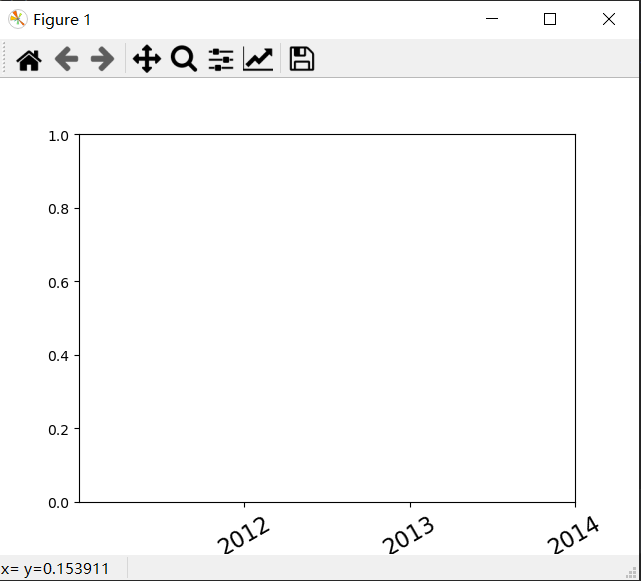参考： https://matplotlib.org/stable/index.html 官方文档 https://blog.csdn.net/mingyuli/article/details/81586380
展开全文• 博客中思维导图的高清PDF版本,可关注公众号 一起学计算机 点击 资源获取 获得 我的更多精彩文章链接, 欢迎查看 经典动漫全集目录 精彩剧集 海贼王 动漫 全集目录 分章节 精彩打斗剧集 思维导图整理 火影忍者 动漫 ...twinx Python
• Matplotlib设置坐标轴日期格式 绘制双坐标轴 ax2=ax1.twinx() 创建了一个独立的Y轴，共享了X轴。双坐标轴！ 类似的还有twiny() 设置坐标轴日期格式 需要导入matplotlib下的dates： import matplotlib.dates ...
• 转载请注明：虚幻私塾 » matplotlib 设置坐标轴2 这次会说到在我们如何移动matplotlib 中 axis 坐标轴的位置. 设置不同名字和位置 使用import导入模块matplotlib.pyplot，并简写成plt 使用import导入模块numpy，并...
• 1.刻度定位器与格式(Tick Locator) ...2.获取坐标轴刻度对象 Matplotlib 中的 ticker 模块用于支持坐标轴刻度的修改，调用下列命令可以初步查看主副坐标轴刻度的数值定位方式（locator）与具体格式（for...plt axis
• import matplotlib.pyplot as plt x = np.linspace(-np.pi,np.pi,50) plt.rcParams['axes.unicode_minus'] = False plt.figure(figsize=(9,6)) plt.plot(x,np.sin(x),x,np.cos(x)) ax = plt.gca() # 获取当前视图 ...python 可视化
• 当日期数据作为图表的坐标轴时通常需要特殊处理，尤其是显示股票数据信息的时候 matplotlib下的dates模块主要用于处理日期 引用库 from matplotlib.dates import DateFormatter, WeekdayLocator, DayLocator, MONDAY...
• ## matplotlib坐标轴调整

千次阅读 2018-10-18 10:42:48
默认画图 from scipy.stats import norm import matplotlib.pyplot as plt import numpy as np x = np.arange(-3, 3, 0.001) plt.plot(x, norm.pdf(x)...对坐标轴进行调整 axes = plt.axes() axes.set_xlim([-5, 5...
• 博客中思维导图的高清PDF版本,可关注公众号 一起学计算机 点击 资源获取 获得 我的更多精彩文章链接, 欢迎查看 经典动漫全集目录 精彩剧集 海贼王 动漫 全集目录 分章节 精彩打斗剧集 思维导图整理 火影忍者 动漫 ...Python
• 在使用matplotlib模块时画坐标图时，往往需要对坐标轴设置很多参数，这些参数包括横纵坐标轴范围、坐标轴刻度大小、坐标轴名称等 xlim():设置x坐标轴范围 ylim():设置y坐标轴范围 xlabel():设置x坐标轴名称 ylabel...
• 前面我们学习过坐标轴显示数字、显示弧度、显示角度、显示日期，如果还想自己搞一些特殊的标签显示，又需要怎么办呢？本文将通过例子来演示怎么样实现这样的功能。另外，也需要考虑坐标轴上显示刻度的数量，有时候...python
• 在机器学习中经常会使用Sigmoid函数，如果直接使用matplotlib绘图，那么就会像下图这样，原点并没有在(0,0)。 import matplotlib.pyplot as plt ...于是，我们希望调整坐标轴的位置，让图像更加的直观
• 坐标轴刻度 import matplotlib as mpl import matplotlib.pyplot as plt import numpy as np plt.style.use('seaborn-whitegrid') %matplotlib inline fig=mpl.figure.Figure() ax=plt.axes() ax.plot(np.random....python
• import matplotlib.pyplot as plt # 1、图形绘制 x = np.linspace(0,2*np.pi) # x # y y = np.sin(x) # 正弦 plt.plot(x,y) # 2、设置xy刻度 plt.xticks(np.arange(0,7,np.pi/2)) plt.yticks([-1,0,1]) #...数据可视化 标签 标题
• matplotlib 可视化 —— 移动坐标轴（中心位置）
• #修改matplotlib中xy字体大小 import matplotlib.pyplot as plt import numpy as np plt.rcParams['font.sans-serif']=['SimHei'] # 用来正常显示中文标签 plt.rcParams['axes.unicode_minus']=False #显示英文 ...python
• 通过spines命令移动横纵坐标轴 ##matplotlib画图初步，代码抄自莫凡大神，地址： ##https://morvanzhou.github.io/tutorials/data-manipulation/plt/2-3-axis1/ import matplotlib.pyplot as plt import numpy as ...
• 当我们要绘制含有多个子图的画布时，很可能遇见需要调整坐标轴的下标排列、范围、是否透明等情况 plt.subplot(121) plt.subplot(122) plt.show() 上图为画两个子图时默认坐标轴 要对坐标轴进行调整，首先得...
• 由于总量数据过大，不太适合与...可以返回画布对象matplotlib.figure.Figure，以及子图的坐标轴对象matplotlib.axes._subplots.AxesSubplot。 plt.subplots(2,3,figsize=(10,8)) 画了一个画布，并且自动按照2行 * 3列
• xsticks和ysticks函数的作用都是获取或设置坐标轴的刻度及标签。其中 xsticks函数作用是获取或设置x坐标轴的刻度及标签。 ysticks函数作用是获取或设置y坐标轴的刻度及标签。 两者参数相同，仅功能稍有不同。 ...标签 ticks
• 设置axes脊柱(坐标系) 属性列表 (1)去掉脊柱(坐标系) ax.spines[‘top’].set_visible(False) #去掉上边框 ax.spines[‘bottom’].set_visible(False) #去掉下边框 ax.spines[‘left’].set_visible(False) #去掉...
• import numpy as np import matplotlib.pyplot as plt x = np.linspace(-np.pi,np.pi,100) data1 = np.exp(x) ...ax1 = plt.gca() # 获取当前域 ax1.set_xlabel('time(s)') # 设置x标签 ax1.spython 数据可视化
• 设置背景色，通过plt.subplot()方法传入facecolor参数，来设置坐标轴的背景色 plt.subplot(facecolor= 'cyan' ); plt.plot(np.random.randn( 10 ),np.arange( 1 , 11 )) 线型 线型 线条...
• import matplotlib.pyplot as plt import numpy as np x = np.linspace(-1,2,100) y1 = 2*x + 1 y2 = x**2 #xy 的范围 plt.xlim((-1,2)) plt.ylim((-2,3)) #xy 描述 plt.xlabel('X') plt.ylabel('Y') #绘制y1 y2两...
• matplotlib库和MATLAB的数据可视化功能几乎是相同，只是因为人工智能的火爆以及使用方便深受大家喜爱。 1.1figure图像 绘制单一函数的图像 import numpy as np import matplotlib.pyplot as plt x = np.linspace(0,...python numpy
• 虽然 Matplotlib 默认的坐标轴定位器（locator）与格式生成器（formatter）可以满足大部分需求，但是并非对每一幅图都合适。此次我将通过一些示例演示如何将坐标轴刻度调整为你需要的位置与格式。 在介绍示例之前，...python 定位 数据分析...